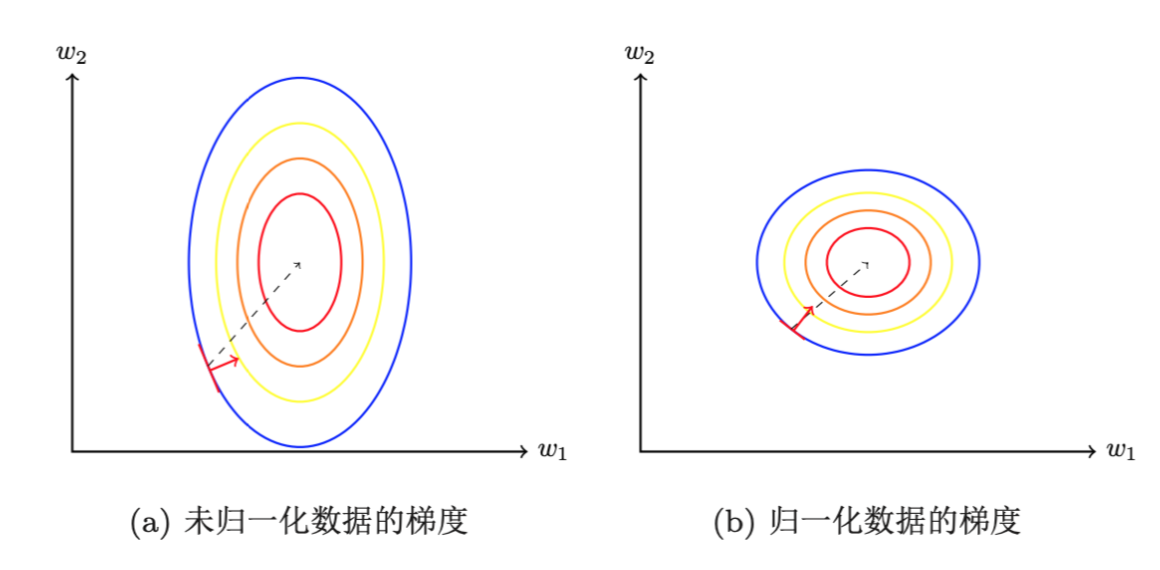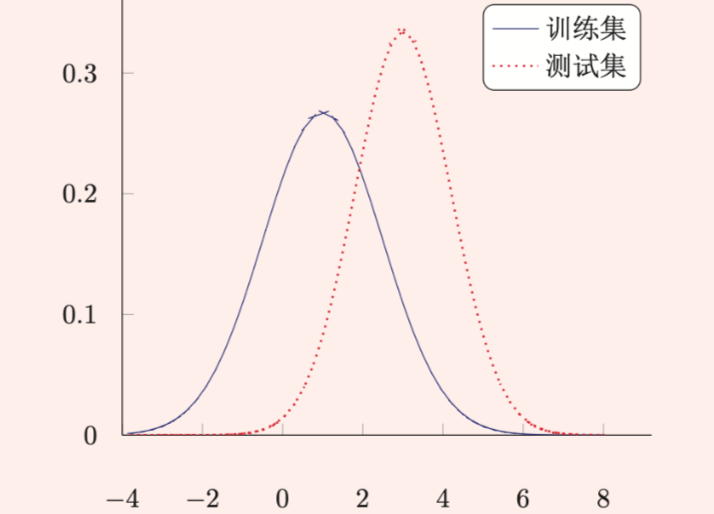# 神经网络中的网络优化和正则化（二）之参数初始化/数据预处理/逐层归一化

Github：https://github.com/thinkgamer

• 第一篇包括
• 网络优化和正则化概述
• 优化算法介绍
• 第二篇包括
• 参数初始化
• 数据预处理
• 逐层归一化
• 第三篇包括
• 超参数优化
• 第四篇包括
• 网络正则化

### 参数初始化

#### 对称权重现象

https://blog.csdn.net/Gamer_gyt/article/details/89440152

#### 均匀分布初始化

Xavier初始化方法是一种自动计算超参数r的方法，参数可以在[-r,r]之间采用均匀分布进行初始化。

$r = \sqrt{ \frac{6}{ n^{l-1} + n^l}}$
$n^l$ 表示第l层神经元的个数，$n^{l-1}$表示l-1层神经元的个数。

$r =4 \sqrt{ \frac{6}{ n^{l-1} + n^l}}$

### 数据预处理

#### 为什么要进行数据预处理### 逐层归一化

#### 深层神经网络中为什么要做逐层归一化• 批量归一化
• 层归一化
• 其他方法

#### 批量归一化

$\hat{z}^l = \frac{ z^l - E[z^l] }{ \sqrt{var(z^l) + \epsilon } }$
$E[z^l]，var(z^l)$表示在当前参数下$z^l$的每一维在整个训练集上的期望和方差，因为深度神经网络采用的是下批量的梯度下降优化方法，基于全部样本计算期望和方差是不可能的，因为通常采用小批量进行估计。给定一个包含K个样本的集合，第$l$层神经元的净输入$z^{(1,l)},....z^{(K,l)}$的均值和方差为：
$\mu _\beta = \frac{1 }{ K } \sum_{k=1}^{K } z^{(k,l)} \\ \sigma^2 _\beta = \frac{1 }{ K }\sum_{ k=1}^{K} (z^{(k,l)} - \mu _\beta)^2$

$\hat{z}^l = \frac{ z^l - \mu _\beta }{ \sqrt{\sigma^2 _\beta+ \epsilon } } \odot \gamma + \beta$

#### 层归一化

$u^l = \frac{1}{n^l} \sum_{i=1}^{ n^l} z_i^l \\ \sigma ^2_l = \frac{1}{n^l} \sum_{i=1}^{ n^l} (z_i^l - u^l )$

$\hat{z^l} = \frac{z^l - u^l }{ \sqrt {\sigma^2 _l + \epsilon } } \odot \gamma + \beta$

$z_t = U h_{t-1} + W x_t \\ h_t = f(\hat{z^l})$

#### 其他方法

• 权重归一化(Weight Normalization)
• 局部响应归一化【搜索与推荐Wiki】专注于搜索和推荐系统，尝试使用算法去更好的服务于用户，包括但不局限于机器学习，深度学习，强化学习，自然语言理解，知识图谱，还不定时分享技术，资料，思考等文章！

0 个人打赏©️2019 CSDN 皮肤主题: 大白 设计师: CSDN官方博客